## The Simple Pendulum

An ideal simple pendulum consists of a point-mass suspended by a flexible, inelastic and weightless string from a rigid support of infinite mass.

In practice, a simple pendulum is obtained by suspending a small metal bob by a long and fine cotton thread about a rigid support.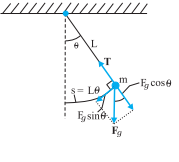Fig. Force acting on the bob of a pendulum

### Expression for time period:

In the equilibrium position, the bob of a simple pendulum lies vertically below the point of suspension. If the bob is slightly displaced on either side and released, it begins to oscillate about the mean position.

1. The force acting on the bob is the force T tension in the spring and the gravitational force Fg (mg).
2. The string makes an angle θ with the vertical.
3. We resolve the force Fg into a radial component Fg cosθ and a tangential component Fg sinθ.
4. The radial component is canceled by the tension since there is no motion along the length of the string.
5. The tangential component produces a restoring torque about the pendulum’s pivot point. This torque always acts opposite to the displacement of the bob so as to bring it back towards its central location.
6. The central location is called the equilibrium position (θ = 0), because at this position the pendulum would be at rest if it were not swinging.
7. The restoring torque τ is given by,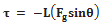where the negative the negative sign indicates that the torque acts to reduce θ, and L is the length of the moment arm of the force Fg sin θ about the pivot point.

### For rotational motion,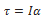Where I is the pendulum’s rotational inertia about the pivot point and α is its angular acceleration about that point.

Above two equation,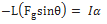Substituting the magnitude of Fg i.e. mg, we have,If we assume that the displacement θ is small. where θ is in radians. So, sinθ can be approx. by θ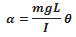The motion of a simple pendulum swinging through small angles is approximately SHM.

• The angular frequency of the pendulum is,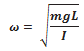• The period of the pendulum, T, is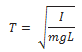All the mass of a simple pendulum is centered in the mass m of the bob, which is at a radius of L from the pivot point. Therefore, for this system, we can write I = mL2

Put it in the equation of time: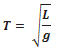It represents a simple expression for the time period of a simple pendulum.